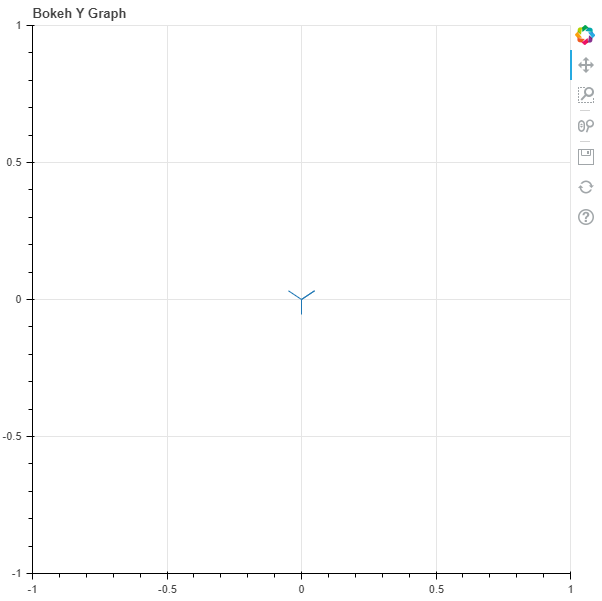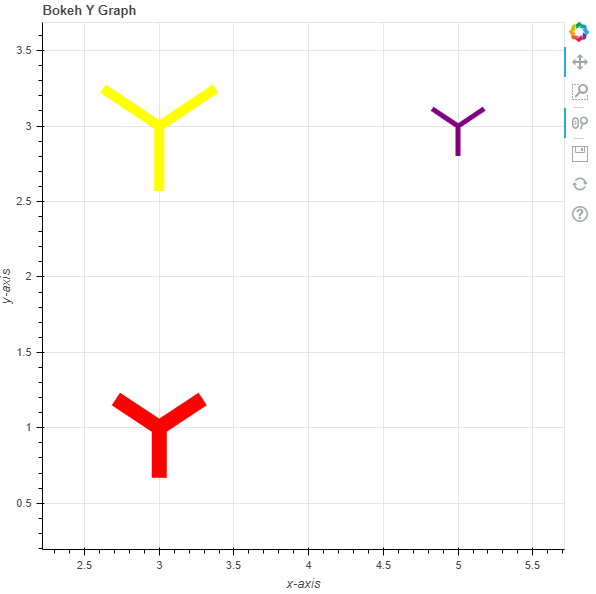# Python Bokeh – Plotting Ys on a Graph

Bokeh is a Python interactive data visualization. It renders its plots using HTML and JavaScript. It targets modern web browsers for presentation providing elegant, concise construction of novel graphics with high-performance interactivity.

Bokeh can be used to plot Ys on a graph. Plotting Ys on a graph can be done using the `y()` method of the `plotting` module.

## plotting.figure.y()

Syntax : y(parameters)

Parameters :

• x : x-coordinates of the center of the y markers
• y : y-coordinates of the center of the y markers

Returns : an object of class `GlyphRenderer`

Example 1 : In this example we will be using the default values for plotting the graph.

 `# importing the modules ` `from` `bokeh.plotting ``import` `figure, output_file, show ` `        `  `# file to save the model ` `output_file(``"gfg.html"``) ` `        `  `# instantiating the figure object ` `graph ``=` `figure(title ``=` `"Bokeh Y Graph"``) ` `      `  `# the points to be plotted ` `x ``=` `0` `y ``=` `0` `     `  `# plotting the graph ` `graph.y(x, y, size ``=` `30``) ` `      `  `# displaying the model ` `show(graph) `

Output :Example 2 : In this example we will be plotting multiple Ys with various other parameters.

 `# importing the modules  ` `from` `bokeh.plotting ``import` `figure, output_file, show  ` `  `  `# file to save the model  ` `output_file(``"gfg.html"``)  ` `       `  `# instantiating the figure object  ` `graph ``=` `figure(title ``=` `"Bokeh Y Graph"``)  ` `  `  `# name of the x-axis  ` `graph.xaxis.axis_label ``=` `"x-axis"` `       `  `# name of the y-axis  ` `graph.yaxis.axis_label ``=` `"y-axis"` `  `  `# points to be plotted ` `x ``=` `[``3``, ``3``, ``5``] ` `y ``=` `[``3``, ``1``, ``3``] ` `size ``=` `[``130``, ``100``, ``60``] ` `  `  `# color value of the Ys ` `color ``=` `[``"yellow"``, ``"red"``, ``"purple"``] ` `  `  `# fill alpha value of the Ys ` `fill_alpha ``=` `[``0.9``, ``0.7``, ``0.5``] ` ` `  `# line width of the Ys ` `line_width ``=` `[``10``, ``15``, ``5``] ` ` `  `# plotting the graph  ` `graph.y(x, y, ` `        ``size ``=` `size, ` `        ``color ``=` `color, ` `        ``fill_alpha ``=` `fill_alpha, ` `        ``line_width ``=` `line_width)  ` `       `  `# displaying the model  ` `show(graph) `

Output :My Personal Notes arrow_drop_upCheck out this Author's contributed articles.

If you like GeeksforGeeks and would like to contribute, you can also write an article using contribute.geeksforgeeks.org or mail your article to contribute@geeksforgeeks.org. See your article appearing on the GeeksforGeeks main page and help other Geeks.

Please Improve this article if you find anything incorrect by clicking on the "Improve Article" button below.

Article Tags :

Be the First to upvote.

Please write to us at contribute@geeksforgeeks.org to report any issue with the above content.